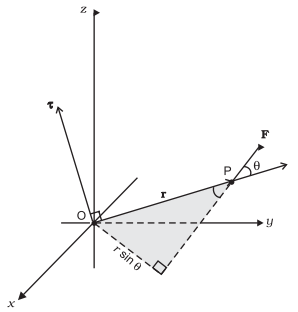The motion of a rigid body, in general, is a combination of rotation and translation. If the body is fixed at a point or along a line, it has only rotational motion. We know that force is needed to change the translational state of a body, i.e. to produce linear acceleration. The rotational analogue of force is the moment of force. It is also referred to as torque.

If a body is capable of rotating about an axis, then force applied properly on this body will rotate it about the axis (axis of rotating). This turning effect of the force about the axis of rotation is called torque.

Torque is the physical quantity which produces angular acceleration in the body.τ = r x F

Torque = the cross-product of displacement r and force F

|τ| = r F sin θ

|τ| = F x r sin θ

But r sin θ = L (momentum arm)

Therefore,

|τ| = F L

Magnitude of Torque = Magnitude of force x Moment Arm

If the line of action of force passes through the axis of rotation then this force cannot produce torque.

Torque → positive if directed outward from paper

Torque → negative if directed inward from paper

The direction of torque can be found by using Right Hand Rule and is always perpendicular to the plane containing r & F. Thus

Clockwise torque → negative

Counter-Clockwise torque → positive

The unit of torque is Nm. Torque is a vector. Moment of force has dimension M L2 T-2. Its dimensions are the same as those of work or energy.

How useful was this post?

Click on a star to rate it!

Average rating 3.1 / 5. Vote count: 9

No votes so far! Be the first to rate this post.

As you found this post useful...

Follow us on social media!

We are sorry that this post was not useful for you!

Let us improve this post!

Tell us how we can improve this post? Please mention your Email so that we can contact you for better feedback.